# How to analyze trends with TREND function in WPS Office Excel

February 18, 2022
498 Views 0

### o Principle of the TREND function

The TREND function predicts the linear increasing amount based on the current data (y=mx+b).

### o Syntax of the TREND function

TREND([known_y's], [known_x's], [new_x's], [logical values])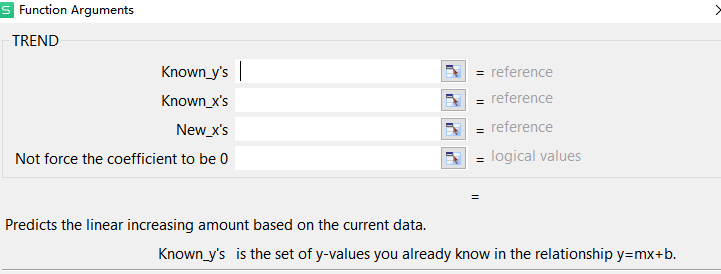### o Explanation of the TREND function

Known_y's (required): the set of y-values you already know in the relationship y=mx+b.

Known_x's (optional): an optional range or array of x-values that you know in the relationship y=mx+b, an array the same size as Know_y’s.

New_x's (optional): a range or array of new x-values for which you want TREND to return corresponding to y-values.

It must have the same number of columns or rows as known_x's.

If omitted, it is assumed to be equal to known_x's.

Logical values(optional): Not force the coefficient to be 0 is a logical value.

The constant b is calculated normally if Const=TRUE or omitted; b set equal to 0 if Const=FALSE.

## · Use the TREND function to analyze trends in a real case

Here we take a sales table as an example. Suppose we want to predict the sales in Beijing and Shanghai in August.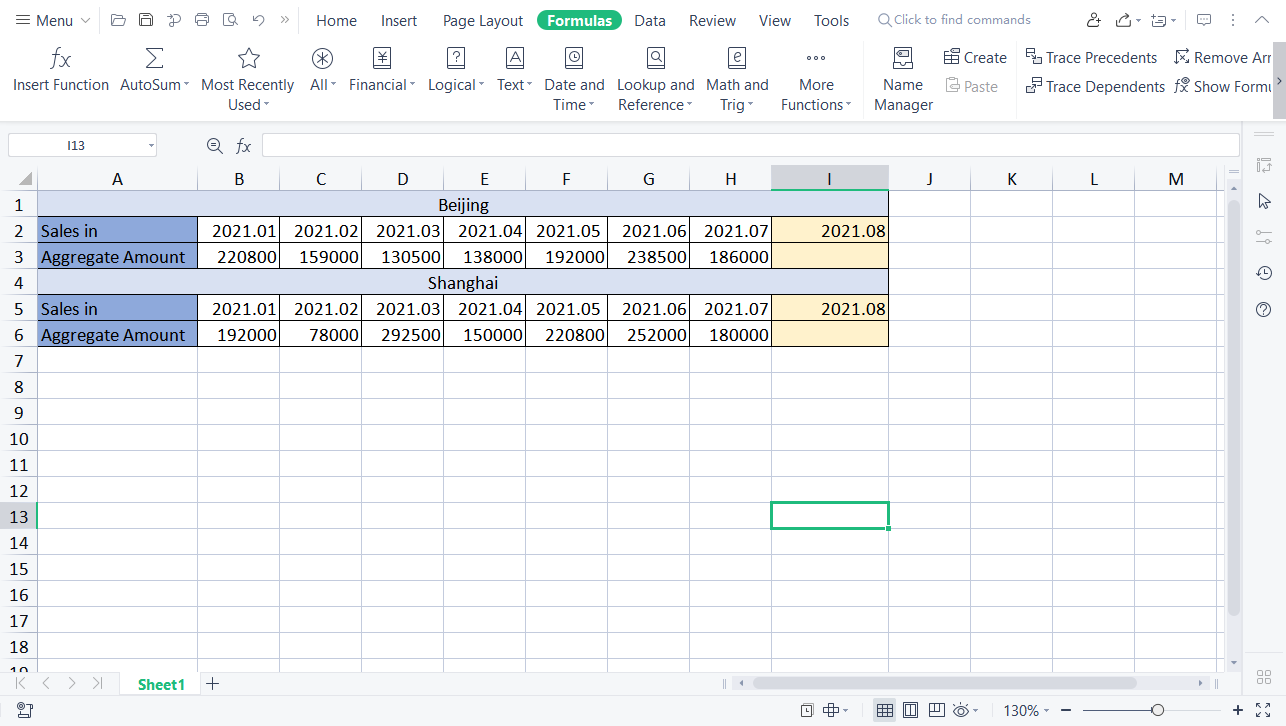1. Select the cell I3, head to the Formulas tab, and click Insert Function.

2. Input “TREND” in the search box and select it.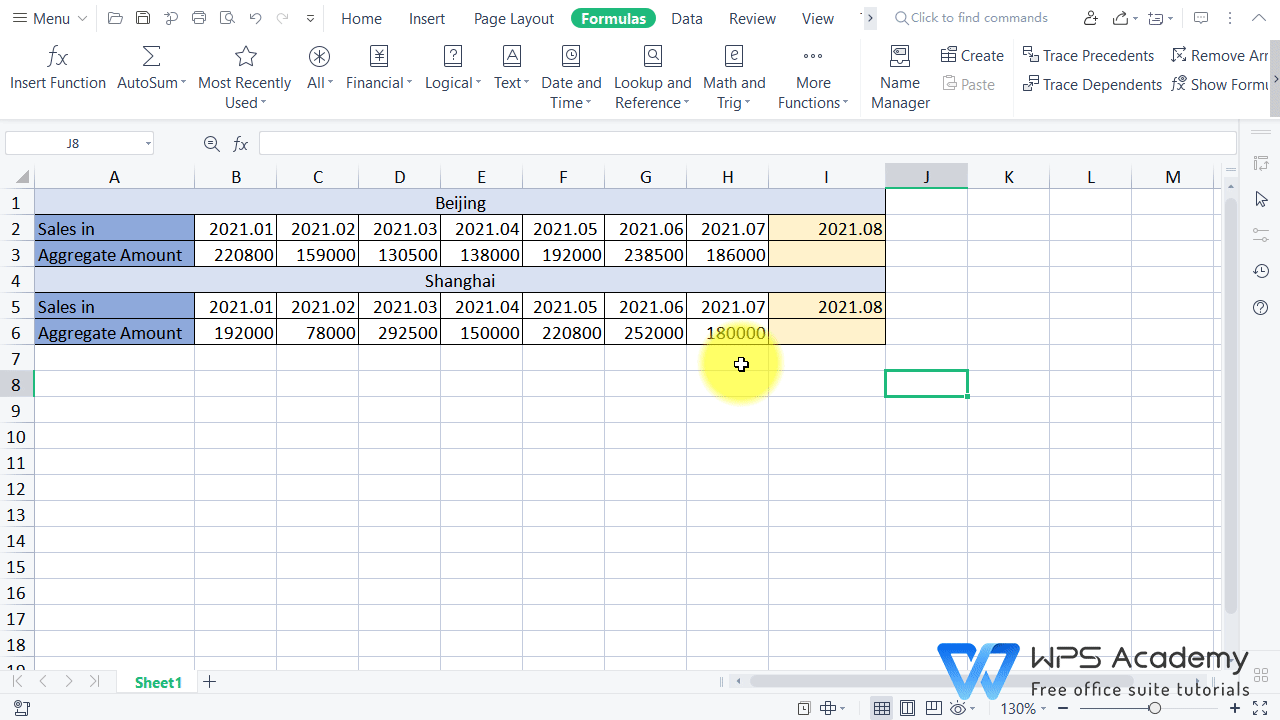3. In this example, select the cell range of B3:H3 at Known_y's, B2:H2 at Known_x's, and I2 at New_x's. We omit Not force the coefficient to be 0 here. Then click OK to return the result.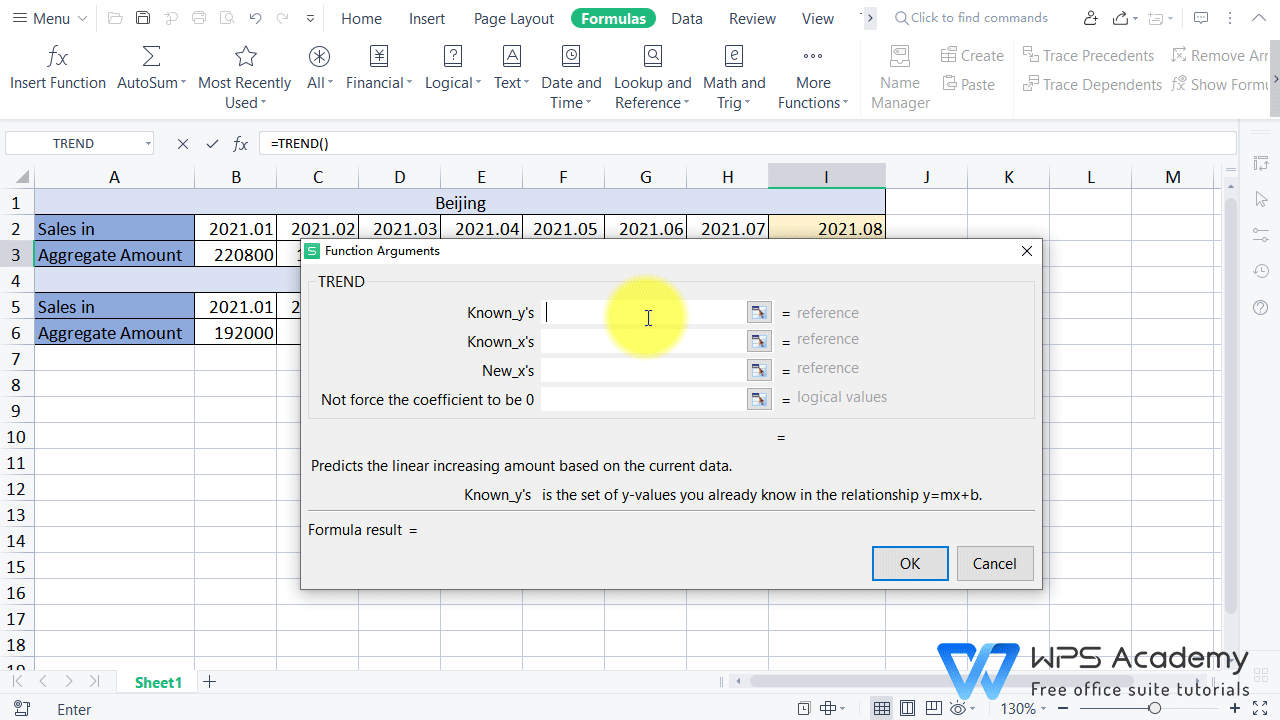4. Then we can repeat the above steps to predict the sales in Shanghai.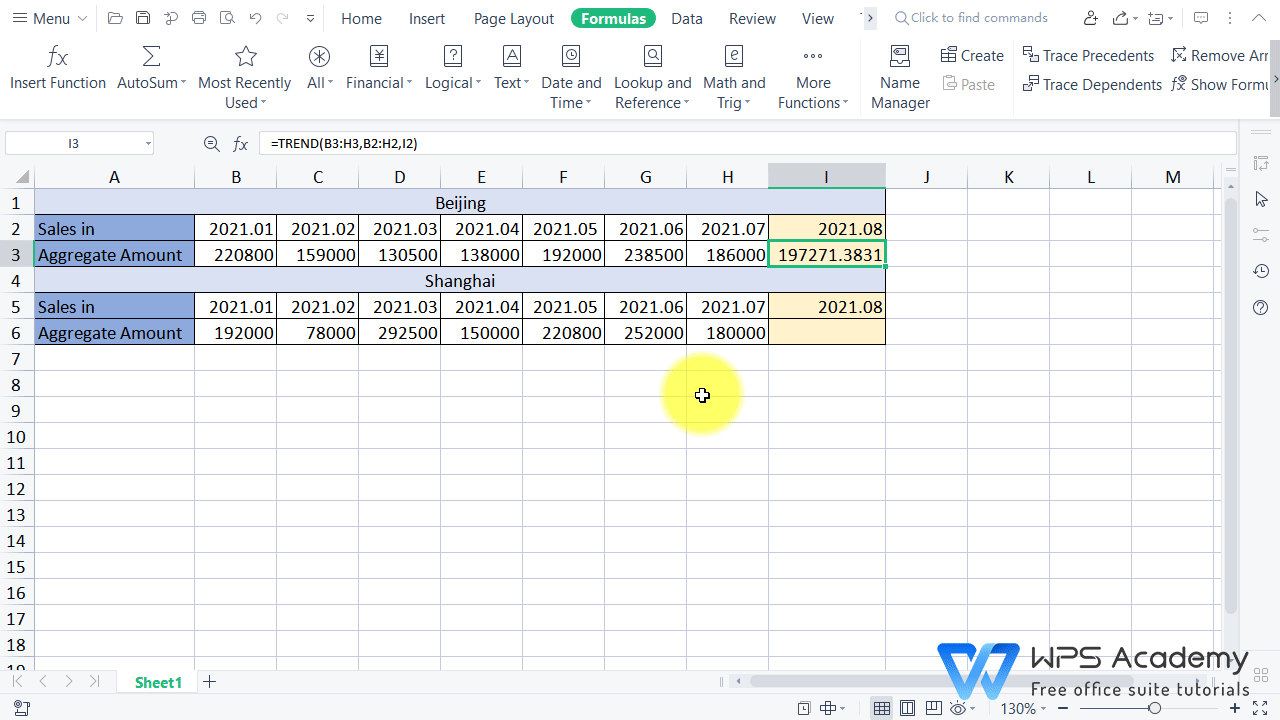With these simple steps, you are able to analyze trends with the TREND function quickly and freely. Did you get it?

## · What is WPS Spreadsheet?

WPS Spreadsheet is a module of WPS Office(an acronym for Writer, Presentation and Spreadsheet). WPS Spreadsheet is consistent with Excel document, which helps users to work with high efficiency. Its personal basic version is free to use and it also provides abundant functions and unique features for you to explore.